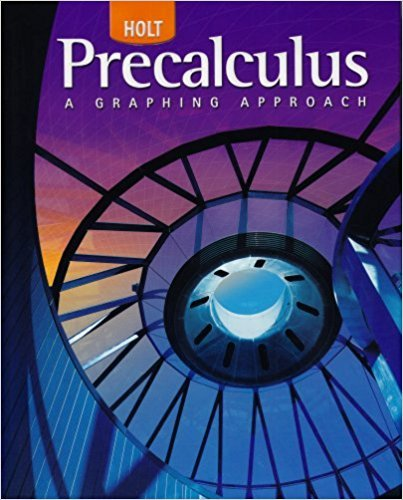×
×

# Solutions for Chapter 11.1: Ellipses## Full solutions for Precalculus | 1st Edition

ISBN: 9780030416477Solutions for Chapter 11.1: Ellipses

Solutions for Chapter 11.1
4 5 0 370 Reviews
16
3
##### ISBN: 9780030416477

Since 25 problems in chapter 11.1: Ellipses have been answered, more than 26049 students have viewed full step-by-step solutions from this chapter. This expansive textbook survival guide covers the following chapters and their solutions. This textbook survival guide was created for the textbook: Precalculus, edition: 1. Precalculus was written by and is associated to the ISBN: 9780030416477. Chapter 11.1: Ellipses includes 25 full step-by-step solutions.

Key Calculus Terms and definitions covered in this textbook
• Algebraic model

An equation that relates variable quantities associated with phenomena being studied

• Cardioid

A limaçon whose polar equation is r = a ± a sin ?, or r = a ± a cos ?, where a > 0.

• Conversion factor

A ratio equal to 1, used for unit conversion

• Coordinate plane

See Cartesian coordinate system.

• Deductive reasoning

The process of utilizing general information to prove a specific hypothesis

• Frequency (in statistics)

The number of individuals or observations with a certain characteristic.

• Head minus tail (HMT) rule

An arrow with initial point (x1, y1 ) and terminal point (x2, y2) represents the vector <8x 2 - x 1, y2 - y19>

• Initial side of an angle

See Angle.

• Interval

Connected subset of the real number line with at least two points, p. 4.

• Magnitude of a real number

See Absolute value of a real number

• Multiplication principle of probability

If A and B are independent events, then P(A and B) = P(A) # P(B). If Adepends on B, then P(A and B) = P(A|B) # P(B)

• NINT (ƒ(x), x, a, b)

A calculator approximation to ?ab ƒ(x)dx

• Observational study

A process for gathering data from a subset of a population through current or past observations. This differs from an experiment in that no treatment is imposed.

• Probability distribution

The collection of probabilities of outcomes in a sample space assigned by a probability function.

• Reference angle

See Reference triangle

• Second-degree equation in two variables

Ax 2 + Bxy + Cy2 + Dx + Ey + F = 0, where A, B, and C are not all zero.

• Standard form of a polynomial function

ƒ(x) = an x n + an-1x n-1 + Á + a1x + a0

A graph in which (-x, y) is on the graph whenever (x, y) is; or a graph in which (-r, -?) or (r, ?, -?) is on the graph whenever (r, ?) is

• Terminal side of an angle

See Angle.

• z-axis

Usually the third dimension in Cartesian space.

×